Created March 1, 2020

# Illuminate - A Smart Night Light

A Smart night light that utilized the smart sense technology to create elegant design and predict user movement.

IntermediateFull instructions provided15 hours22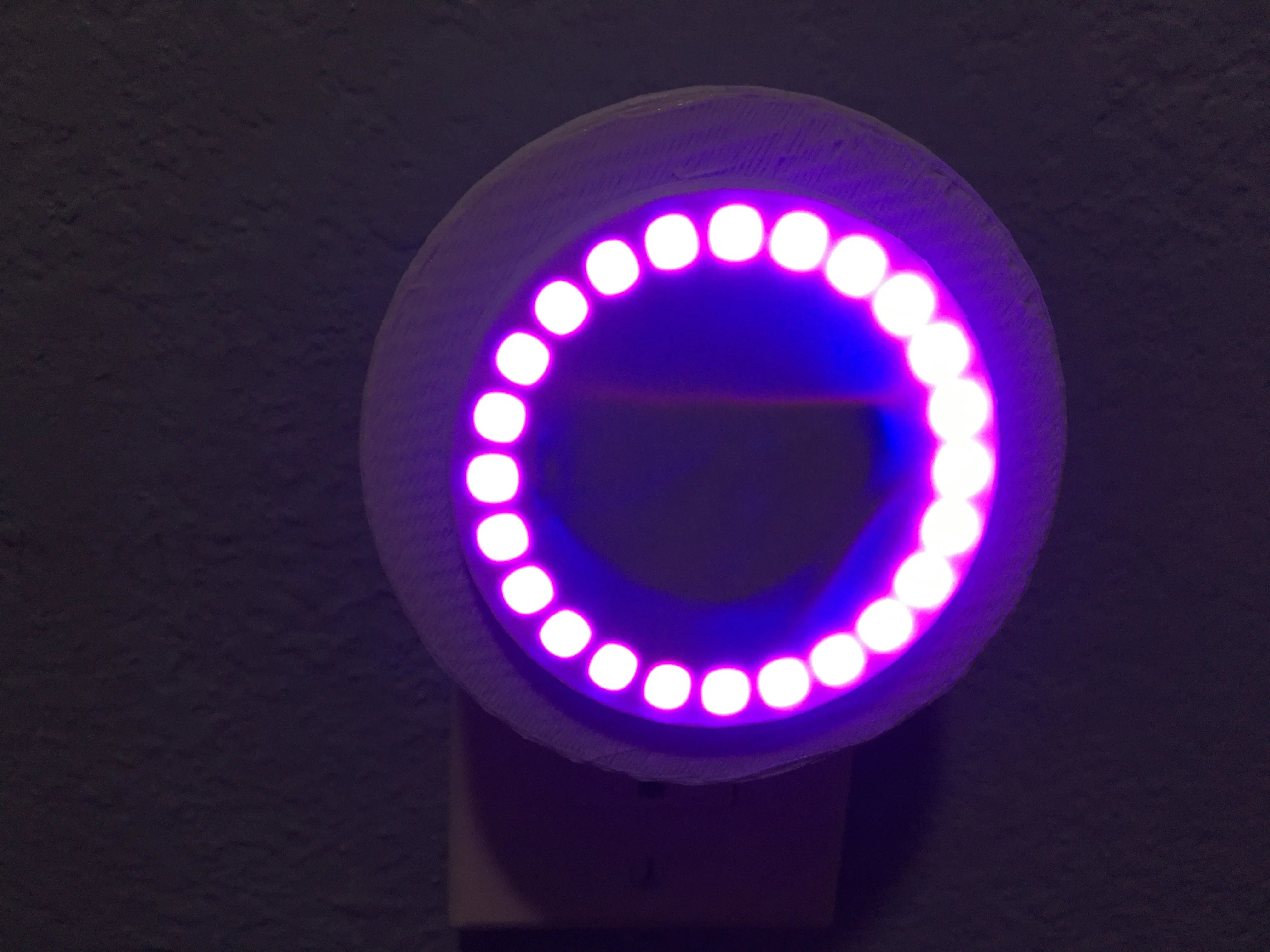## Things used in this project

### Hardware components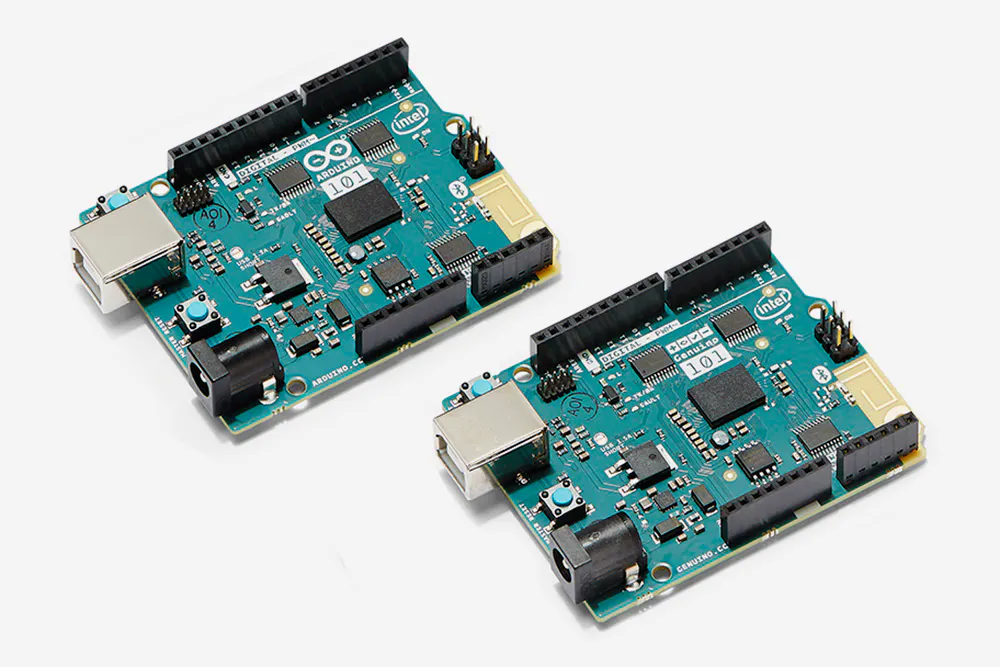Arduino 101 & Genuino 101
×1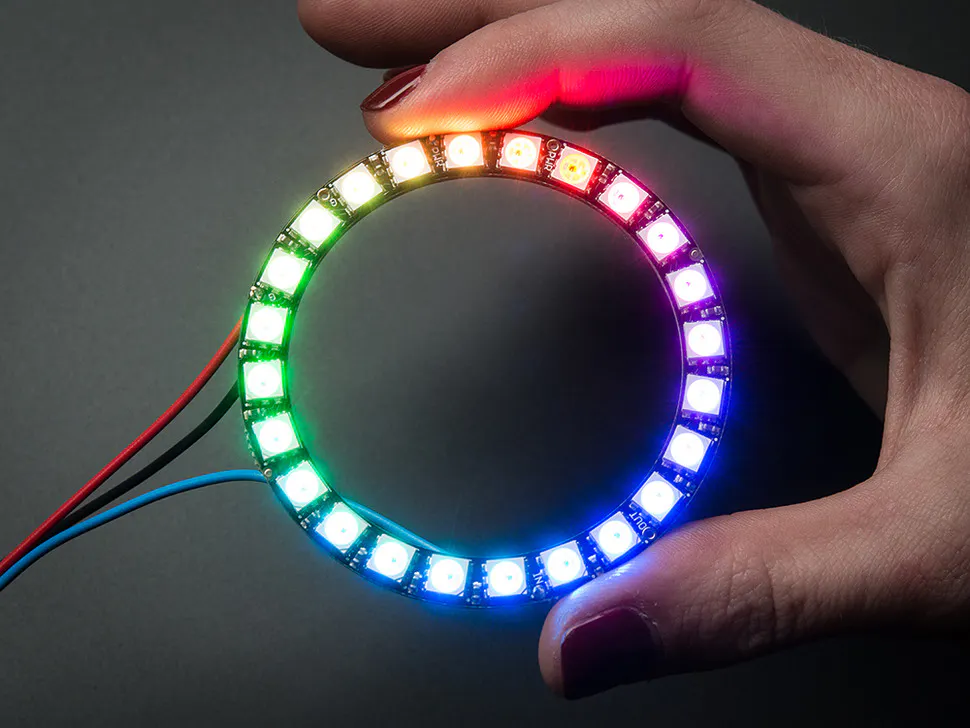Adafruit NeoPixel Ring: WS2812 5050 RGB LED
×1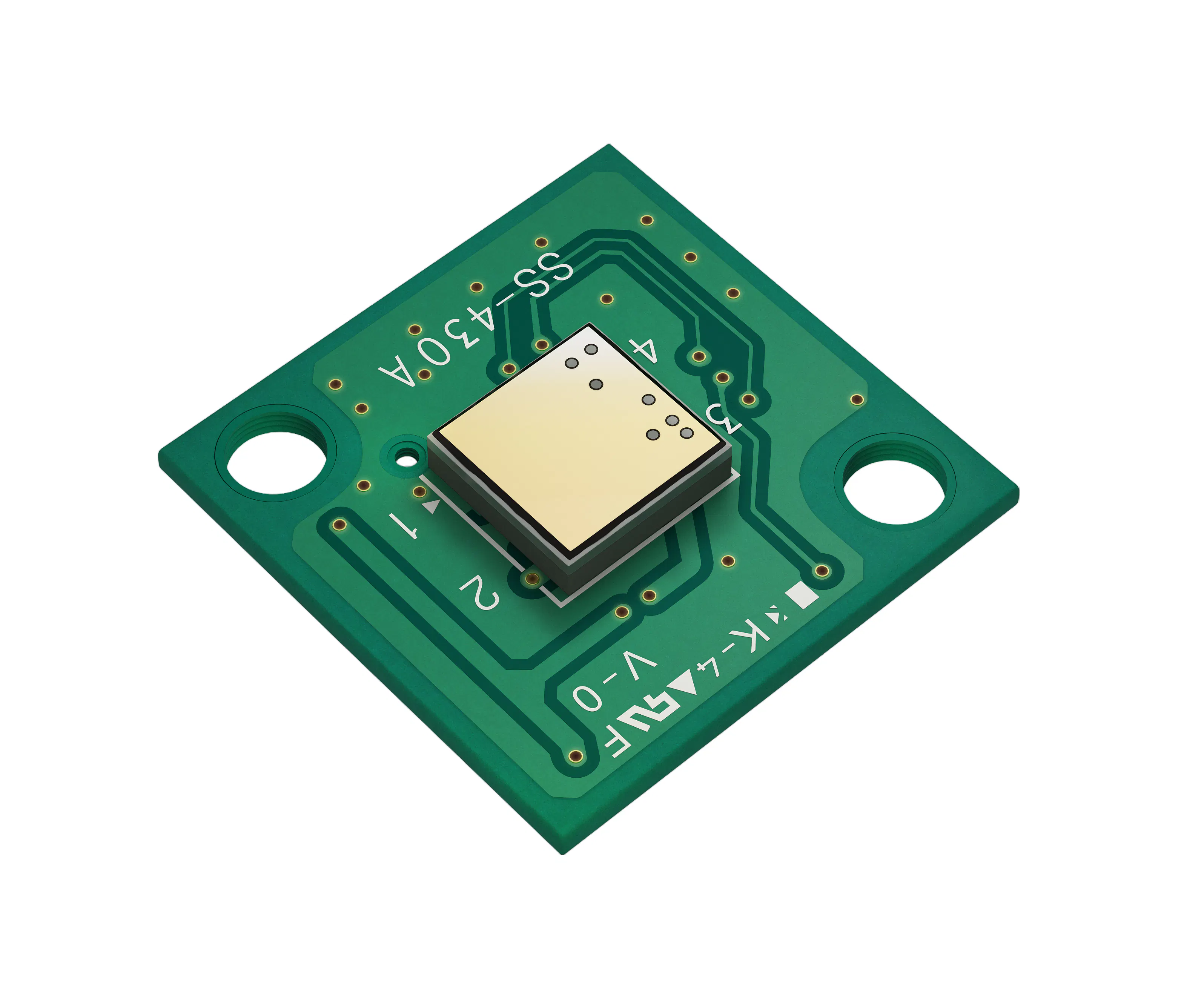KEMET Electronics Corporation Proximity Sensor- Pyroelectric Infrared Sensor Module
×1

## Schematics

### Layout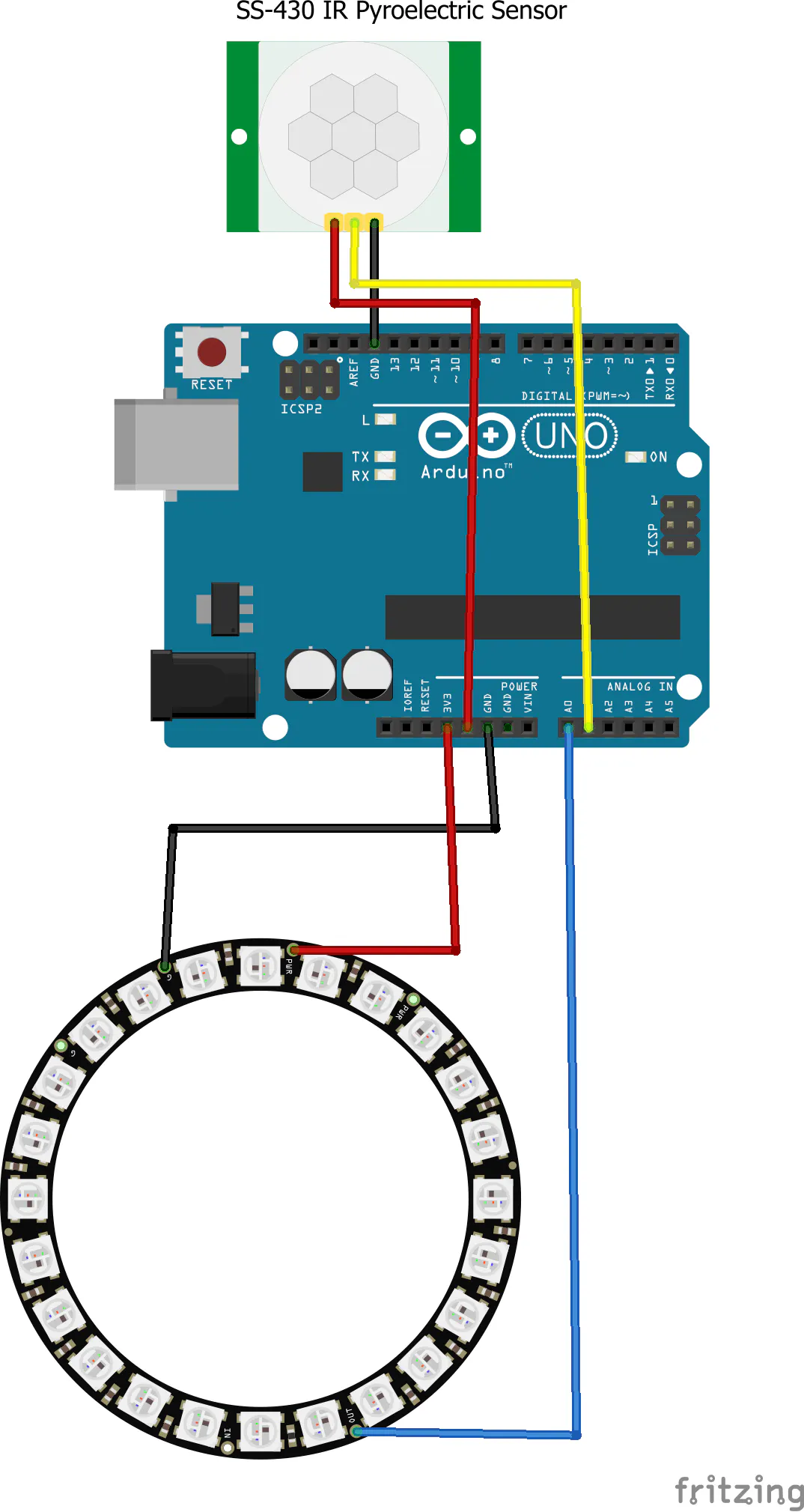## Code

### Arduino Sketch

Arduino
```//#define BLYNK_USE_DIRECT_CONNECT
#include <BlynkSimpleCurieBLE.h>
#include <CurieBLE.h>
#ifdef __AVR__
#include <avr/power.h>
#endif
#define PIN        A0
#define NUMPIXELS 2
#define BLYNK_PRINT Serial
#define MAXIMUM_BRIGHTNESS 255
#define MEDIUM_BRIGHTNESS  130

BLEPeripheral  blePeripheral;

// You should get Auth Token in the Blynk App.
// Go to the Project Settings (nut icon).
char auth[] = "KgvVFbtQXaUkINbxWslpJMObn8Fw2X4L";

int Brightness; // variable to change brightness
int ColourNumber;
int red;   //
int green; // variables for custom colour
int blue;  //
int maxBrightness = 150;
int minBrightness = 3;
int ispulsing = 0;
int poweroff = 0;
int Pyro = A1;
int counter = 0;
unsigned long IR_threshold = 198000;

// Note: SS-430 has two pulses of 200msec per detection.
// IR_threshold is in microsec (usec), therefore 198msec threshold
int IR_sensed = 0;

//Variables
int value;          // Store value from photoresistor (0-1023)
int buttonvalues; //Define a numeric variable

void setup(){
Serial.begin(9600);
pixels.begin();
pixels.setBrightness(10);
pixels.show();

pinMode(buttonpin,INPUT);
pinMode(SS430, INPUT);// Set SS430 - A1 pin as an input

delay(1000);

blePeripheral.setLocalName("Kemet");
blePeripheral.setDeviceName("Kemet");
blePeripheral.setAppearance(384);
Blynk.begin(auth, blePeripheral);
blePeripheral.begin();

}

void loop(){
Blynk.run();
blePeripheral.poll();

PyroRead = pulseIn(A1, HIGH); //Measure trigger point
if(PyroRead > IR_threshold){ //Make sure trigger is over 198msec)
pulse();
}
delay(50); //Small delay
}

// Fill the dots one after the other with a color
void colorWipe(uint32_t c, uint8_t wait) {
for (uint16_t i = 0; i < pixels.numPixels(); i++) {
pixels.setPixelColor(i, c);
pixels.show();
delay(wait);
}
}

void pulse() {
for (int x = minBrightness; x < MAXIMUM_BRIGHTNESS; x++) {
pixels.setBrightness(x);
pixels.show();
delay(10); //Small delay

}
for (int x = MAXIMUM_BRIGHTNESS; x >= minBrightness; x--) {
pixels.setBrightness(x);
pixels.show();
delay(10); //Small delay

}
}

BLYNK_WRITE(V4) //read slider value for red
{
int pinValue = param.asInt(); // assigning incoming value from pin V4 to a variable
// You can also use:
// String i = param.asStr();
// double d = param.asDouble();
Serial.print("red Slider value is: ");
Serial.println(pinValue);
red = map(pinValue, 0, 1023, 0, 255);
}

BLYNK_WRITE(V5) //read slider value for green
{
int pinValue = param.asInt(); // assigning incoming value from pin V5 to a variable
// You can also use:
// String i = param.asStr();
// double d = param.asDouble();
Serial.print("green Slider value is: ");
Serial.println(pinValue);
green = map(pinValue, 0, 1023, 0, 255);
}

BLYNK_WRITE(V6) //read slider value for blue
{
int pinValue = param.asInt(); // assigning incoming value from pin V6 to a variable
// You can also use:
// String i = param.asStr();
// double d = param.asDouble();
Serial.print("blue Slider value is: ");
Serial.println(pinValue);
blue = map(pinValue, 0, 1023, 0, 255);
}

{
int pinValue = param.asInt(); // assigning incoming value from pin V2 to a variable
Serial.print("Button value: ");
Serial.println(pinValue);
switch (pinValue) {
case 1: //white
{
colorWipe(pixels.Color(255, 255, 255), 50); // White
break;
}

case 2: //purple
{
colorWipe(pixels.Color(106, 13, 173), 50); // White
break;
}

case 3://yellow
{
colorWipe(pixels.Color(250, 218, 94), 50); // White
break;
}

case 4://Turquoise
{
colorWipe(pixels.Color(0, 255, 239), 50); // White
break;
}

case 5://Custom
{
colorWipe(pixels.Color(red, green, blue), 50); // White
break;
}
}
}

{
int pinValue = param.asInt(); // assigning incoming value from pin V1 to a variable
// You can also use:
// String i = param.asStr();
// double d = param.asDouble();
Serial.print("V1 Slider value is: ");
Serial.println(pinValue);
Brightness = map(pinValue, 0, 1023, 1, 255);
pixels.setBrightness(Brightness);
pixels.show();
}

{
int pinValue = param.asInt(); // assigning incoming value from pin V1 to a variable
// You can also use:
// String i = param.asStr();
// double d = param.asDouble();
Serial.print("V0 is: ");
Serial.println(pinValue);
ispulsing = pinValue;
}

{
int pinValue = param.asInt(); // assigning incoming value from pin V1 to a variable
poweroff = pinValue;
}